## Indicators

•Cited by SciELO
•Access statistics

•Cited by Google
•Similars in SciELO
•Similars in Google

## Print version ISSN 0123-921X

### Tecnura vol.23 no.60 Bogotá Apr./June 2019

#### http://dx.doi.org/10.14483/22487638.14929

Estudio de caso

Darcy-Weisbach resistance coefficient determination using Newton-Raphson approach for android 4.0

Determinación del coeficiente de fricción de Darcy-Weisbach mediante la utilización del método de aproximación Newton-Raphson para aplicaciones Android 4.0

Edgar Orlando¡no Lad Moreno1  , César Augusto García Ubaque2  , María Cam¡la García Vaca3

1 Civil Engineer, Topographic Engineer, MsC Civil Engineering. Associated Professor Universidad Militar Nueva Granada, Bogotá D. C. Contact: edgar.ladino@unimilitar.edu.co. ORCID: https://orcid.org/0000-0002-7770-452X.

2 Civil Engineer, MsC Civil Engineering, PhD in Engineering. Associated Professor Universidad Distrital Francisco José de Caldas, Bogotá D. C. Contact: cagarciau@udistrital.edu.co. ORCID: https://orcid.org/0000-0002-6959-6610.

3 Chemical Engineer, MsC Chemical Engineering. Colombia, Bogotá D. C. Contact: mcgarciav@ucatolica.edu.co. ORCID: https://orcid.org/0000-0002-1382-7387.

Abstract

Context:

The art¡cle presents the fr¡ct¡on coeff¡c¡ent calculat¡on for pressure p¡pes, from Newton-Raphson numer¡cal method, under JavaScr¡pt source code for Andro¡d 4.0 or h¡gher.

Methodology:

The code development ¡s based on Colebrook-Wh¡te model for turbulent flows. The app also establ¡shes the fr¡ct¡on coeff¡c¡ent for lam¡nar flows from the Po¡seu¡lle equat¡on and determ¡nes the range for the enter flow ¡n a trans¡t¡on zone (unstable flow). An algor¡thm of success¡ve approx¡mat¡ons was ¡mplemented for non-l¡near equat¡on solut¡on based on the relat¡ve roughness and Reynolds number.

Results:

In the Newton-Raphson method, ¡f the ¡n¡t¡al value moves away from zero or presents d¡fferent ¡nflect¡on po¡nts, the probab¡l¡ty of d¡vergence of the method ¡ncreases s¡gn¡f¡cantly. To overcome th¡s anomaly, a fr¡ct¡on coeff¡c¡ent equal to 0.015 was proposed as ¡n¡t¡al (seed) value; thereby we guarantee the convergence for turbulent flows w¡th an approx¡mat¡on of 0.0000001. The values calculated by the app were cal¡brated w¡th the Excel analys¡s tool (Goal Seek).

Conclusion:

The app ¡s ava¡lable for free ¡n Google Play Store, and ¡s a tool to support educat¡onal processes and the profess¡onal f¡eld.

Key words: Andro¡d; coeff¡c¡ent of fr¡ct¡on; Colebrook-Wh¡te; Darcy-We¡sbach; head losses ¡n p¡pes; JavaScr¡pt code; Newton-Raphson; numer¡cal methods

Resumen

Contexto:

El artículo presenta el cálculo de coef¡c¡ente de fr¡cc¡ón para tubos de pres¡ón, ut¡l¡zando el método numér¡co Newton-Raphson, usando cód¡go JavaScr¡pt para Andro¡d 4.0 o super¡or.

Metodología:

El desarrollo del Cód¡go está basado en el modelo de Colebrook-Wh¡te para flujos turbulentos. La apl¡cac¡ón tamb¡én establece el coeficiente de fricción para flujos laminares utiliando la ecuación de Poiseuille, y determina el rango de flujo de entrada en zona de trans¡c¡ón (flujo ¡nestable). Se ¡mplementó un algor¡tmo de aprox¡mac¡ones suces¡vas para soluc¡ón de ecuac¡ones no lineales basado en la rugosidad relativa y en el número de Reynolds.

En el método de Newton-Raphson, la probabilidad de divergencia aumenta considerabemente si el valor inicial es diferente de cero o presenta distintos puntos de inflección. Para superar esta anomalía, se propone un coeficiente de fricción de 0.015 como valor inicial (valor semilla). De esta manera se garantiza la convergencia de flujos turbulentos con aproximación de 0.0000001. Los valores calculados por la aplicación fueron calibrados utilizando la herramienta de análisis de Excel© (Goal Seek).

Conclusión:

La aplicación está disponible en Google Play Store y es gratuita. Es una herramienta para apoyar procesos educativos y trabajos en sector productivo.

Palabras-clave: Android; coeficiente de fricción; Colebrook-White; Darcy-Weisbach; tuberías; JavaScript; Newton-Raphson; métodos numéricos

INTRODUCTION

In the last decade, the development of apps for mobile devices on Android platform, along with the increase of processing velocity and storage capacity, have originated user- service oriented technologies. This is especially evident in the case of specialized user needs. An increase of software development for mobile devices to solve specific problems in business (m-business), marketplace (m-commerce), and education (m-learning) has been observed (Gasca, Camargo, & Medina, 2013) (Yin, Weng, & Chu, 2012).

Educative Software Engineering (ESE) classifies these computational tools into algorithms and heuristics. Algorithms pose different systematic processes that lead the student to a determined answer. On the other hand, heuristic tools lead to appropriation of knowledge by means of experimentation and autonomous discovering. Synergies between both algorithmic and heuristic tools produce the intelligent tutorial systems development (Galvis A., 1992).

Therefore, the aim of this paper is to present the development of a mobile app in Android platform that calculate Darcy's friction factor, to be used as a tool in both learning and professional processes. JavaScript source code for Android v. 4.0 or higher was used. Poiseuille model was used for laminar flow cases, and Colebrook-White model for turbulent flow cases. Transition zone between the two types of developed flow regimen, where flow present an unstable behavior, was also calculated.

Friction coefficient

According to regulation for potable water and basic sanitation (RAS 2017), hydraulic calculation for pressured systems in Colombia must be effectuated using Darcy-Weisbach 1845 model. It is a widely known empiric equation that determines load loss due to energy dissipation in the form of friction. This friction is the result of the interaction between fluid and its conducing structure, or pipeline (equation (1)).

Where, h f : load loss (m); f: friction coefficient; L: pipe length (m); D: pipe diameter (m); V: flow velocity (m/s); g gravity acceleration (m/s2).

There are numerous models to calculate Darcy's friction coefficient, or friction factor. Most of them are empirical models limited to the range of experimentation in which they were formulated, such as Moody equation. Another example is Swamee-Jain general equation. It presents satisfactory results for most flow conditions, except for smooth turbulent flow, in which a correction factor is necessary (Andrade, 2001).

However, Colebrook-White model has been widely preferred due to its precision within turbulent conditions. Since it is a non-explicit expression, its solution requires the use of numeric methods. In this work, we used Newton-Rapson method for optimizing point integration (equation (2)).

Where, f: Friction coefficient; e: pipe rugosity (m); D: pipe diameter (m); R e : Reynolds Number.

Laminar Flow

Laminar Flow is determined by values of Reynolds number below 2300. It is known that under this flow condition, viscous forces are significant in comparison to inertial forces, and therefore the fluid moves in an overlapping form with independent layers. In this case, the system behaves according to the Newton equation for viscous fluids. (Saldarriaga, 2007).

In figure 1, we see the typical velocity distribution of a laminar flow inside a cylindric pipe. The highest velocity is reached in the center of the tube, and the lowest flow velocities are found in the surface of the pipe, where the fluid is in direct contact with the solid walls. The result of this phenomenon is a parabolic velocity profile. (Saldarriaga, 2007).

In the condition of a laminar flow, and therefore a Reynolds Number below 2300, friction coefficient may be calculated from Poiseuille equation (equation (3)).

According to Reynolds' early experiments, for values between 2300 and 4000, approximately, the flow undergoes a transitional behavior. Because of that, this range of values of Reynolds Number is known as the Transition Zone. In this case, neither laminar or turbulent flow are well defined, and thus friction factor cannot be calculated (equation (4)).

Turbulent Flow

Turbulent flow may be defined as a vectoral chaos; in it we find continuous mixture conditions, due to vortex rupture. (Sotelo, 1997). Despite this widely observed phenomenon, recent studies have found vortex behavioral patterns in turbulent systems, which may defy the paradigms of turbulence according to current notions. In fact, tests to model disorganized and complex turbulent flow conditions into series of somehow organized movement patterns, known as "coherent structures", have been made with mixed results. (Dennis, 2015).

Newton-Raphson Method

Newton-Raphson method optimizes the Newton point integration method by defining a more robust convergence equation. Convergence will exist if the difference of absolute value of calculated values in two successive iterations decreases while the number of iterations (n) increases (Quinta & Villalobos, 2005). The algorithm has been generalized in numerous forms to solve non-linear problems, equation systems, non-linear differential, and integral equations (Díaz & Benítez, 1998). However, if the function is not differentiable and presents discontinuity in the calculation interval, the Newton-Raphson method will not converge. In such cases, it will be necessary to apply another numeric method to find the root of the non-linear equation. Newton-Raphson method requires an initial assumed value f 0 (seed value) (equation (5)).

Now, in the problem of calculating the friction factor, Colebrook-White equation becomes the function, as noted below (equation (6)).

And, the first derivative becomes (equation (7)).

Therefore, Newton-Rapson convergence equation will become (equation (8)).

METHODOLOGY

In this work, we followed four main methodological steps (figure 2): (1) to define the numeric method to solve Colebrook-White equation in the Turbulent Flow condition, (2) to stablish the flow diagram of the app's iterative process, (3) to develop the source code in JavaScript, and (4) to calibrate resulting calculations with Goal Seek function in Excel.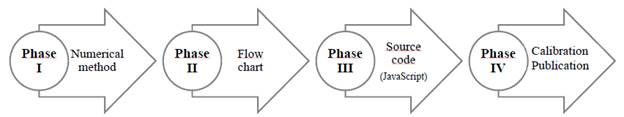Source: own work.

Figure 2 methodological steps followed during the development of the app.

Source code was developed using JavaScript. Once the solution converges, the result appears in a separated window on the screen. The relative rugosity and Reynold Number, which are both dimensionless.

In figure 3, we present the flow diagram of the iterative process the app follows to calculate Darcy's friction factor.

RESULTS

Resulting calculations for Darcy's friction factor were calibrated by comparing them to solutions calculated through the Goal Seek function in Excel. In table 1, we present the comparison between various calculation with different input data.

Laminar flow conditions

The relative rugosity of a pipe is 0.0000015. If the Reynolds Number is 1550, the app conduces the calculation of friction coefficient using Poiseuille model for Laminar Flow. Therefore, the result will be f = 0,0412903, as shown in figure 4.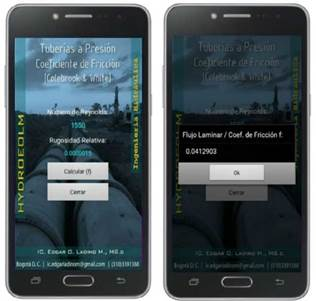Source: own work.

Figure 4 Laminar flow (Friction coefficient app)

Transition zone flow conditions

If the relative rugosity of the pipe is 0.0000045 and the Reynolds Number is 3456, the flow is in the transition zone, so the app will indicate exactly that as shown in figure 5.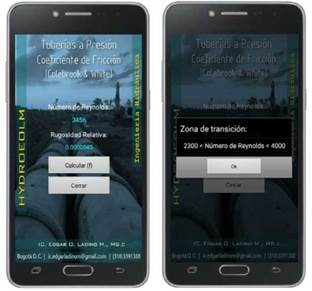Source: own work.

Figure 5 Unstable flow (Friction coefficient app)

Turbulent flow conditions

If the relative rugosity of a pipe is 0.0000018 and the Reynolds Number is 845203, the app will use Newton-Raphson method to solve Colebrook-Whi-te equation and calculate the friction coefficient. Seed value is already determined to f= 0.015. the result is f = 0,0120250, as shown in figure 6.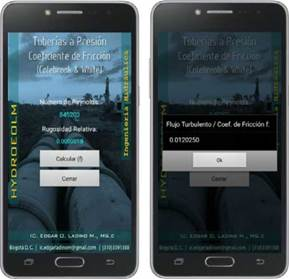Source: own work.

Figure 6 Turbulent Flow (Friction coefficient app)

The solution for turbulent flow conditions is coherent with the one obtained by the Goal Seek function, shown in the table 2.

Table 2 Friction Coefficient calculation through Excel goal seek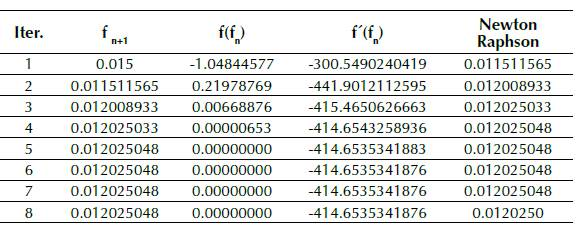Source: own work

CONCLUSIONS

The app "App cálculo del coeficiente de fricción" accurately calculates Darcy's friction factor for Laminar and Turbulent Flow Conditions. It also indicates when the input data corresponds to the Transition Zone.

The development of mobile apps for educational purposes (m-learning) is a valuable tool for autonomous learning. Therefore, the app "App cálculo del coeficiente de fricción" contributes to Fluid Mechanics and Hydraulics academical environments. It allows students to experiment with varied input data and explore different initial conditions of the problem.

REFERENCES

Andrade, L. &. (2001). Analysis of the Swamee-Jain's equation for the calculation of friction factor. Revista Brasileira de Engenharia Agrícola e Ambiental. https://doi.org/10.1590/s1415-43662001000300030Links ]

Dennis, D. J. (2015). Coherent structures in wall-bounded turbulence. Anais da Academia Brasileira de Ciências, 1162. https://doi.org/10.1590/0001-3765201520140622Links ]

Díaz & Benítez, J. M. (1998). Introducción a los métodos numéricos para la resolución de ecuaciones. Cádiz: Ingrasa Artes Gráficas. [ Links ]

Galvis A., P. (1992). Ingeniería de Software Educativo. Bogotá D. C.: Ediciones Uniandes. [ Links ]

Gasca M. C. et al, M. (2013). Metodología para el desarrollo de aplicaciones móviles. Tecnura, 22. [ Links ]

Gómez C. et al, R. (1998). Ingeniería de software educativo con modelaje orientado por objetos: un medio para desarrollar micromundos interactivos. Informática Educativa Uniandes, 37. [ Links ]

Lindley, C. (2013). Java Script Enlightenment. New York: Simon St. Laurent and Meghan Blanchette. [ Links ]

Quinta & Villalobos, P. E. (2005). Métodos Numéricos con aplicaciones en Excel. Barcelona: Reverté S. A. [ Links ]

Saldarriaga, J. (2007). Hidráulica de Tuberías. Bogotá D. C.: Alfaomega. [ Links ]

Sotelo, G. (1997). Hidráulica General. México D. F.: Limusa. [ Links ]

Cómo citar: Lad¡no M., E.O, García U., C.A. y García V., M.C. (2019). Darcy-We¡sbach res¡stance coeff¡c¡ent determ¡nat¡on us¡ng Newton-Raphson approach for andro¡d 4.0. Tecnura, 23(60), 52-58. DOI: https://do¡.org/10.14483/22487638.14929

Received: August 15, 2018; Accepted: February 14, 2019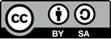Todo el contenido de esta revista, excepto dónde está identificado, está bajo una Licencia Creative CommonsThis is an open-access article distributed under the terms of the Creative Commons Attribution License